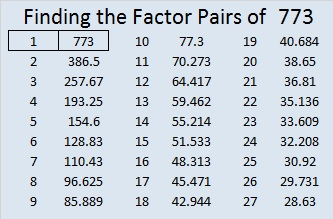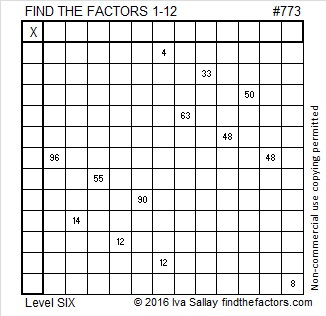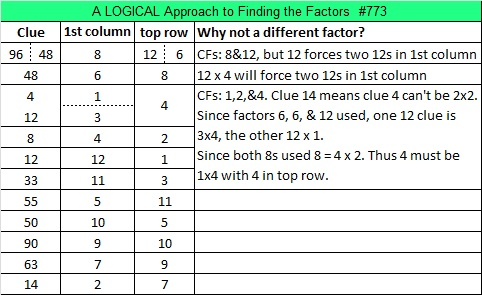# 773 and Level 6

• 773 is a prime number.
• Prime factorization: 773 is prime.
• The exponent of prime number 773 is 1. Adding 1 to that exponent we get (1 + 1) = 2. Therefore 773 has exactly 2 factors.
• Factors of 773: 1, 773
• Factor pairs: 773 = 1 x 773
• 773 has no square factors that allow its square root to be simplified. √773 ≈ 27.8028775.How do we know that 773 is a prime number? If 773 were not a prime number, then it would be divisible by at least one prime number less than or equal to √773 ≈ 27.8. Since 773 cannot be divided evenly by 2, 3, 5, 7, 11, 13, 17, 19, or 23, we know that 773 is a prime number.

Here is today’s puzzle for you to try to solve:Print the puzzles or type the solution on this excel file: 12 Factors 2016-02-25

————————————-

What else is special about the number 773?

22² + 17² = 773 so 773 is the hypotenuse of the primitive Pythagorean triple 195-748-773 which was calculated using 22² – 17², 2(17)(22), 22² + 17².

Thus 195² + 748² + 773².

773 is also the sum of three squares six different ways:

• 26² + 9² + 4² = 773
• 25² + 12² + 2² = 773
• 24² + 14² + 1² = 773
• 23² + 12² + 10² = 773
• 22² + 15² + 8² = 773
• 20² + 18² + 7² = 773

773 is a palindrome in two other bases:

• 545 BASE 12, note that 5(144) + 4(12) + 5(1) = 773
• 3D3 BASE 14 (D = 13 base 10); note that 3(196) + 13(14) + 3(1) = 773

Here’s another way we know that 773 is a prime number: Since  its last two digits divided by 4 leave a remainder of 1, and 22² + 17² = 773 with 22 and 17 having no common prime factors, 773 will be prime unless it is divisible by a prime number Pythagorean triple hypotenuse less than or equal to √773 ≈ 27.8. Since 773 is not divisible by 5, 13, or 17, we know that 773 is a prime number.

————————————-## 2 thoughts on “773 and Level 6”

1.davescarthin

I’ve done a lattice labyrinth for the sufficiently massive prime 773, based on number pair (17,22), 17^2 + 22^2 being equal to 773, as you point out, but don’t know how to send it. Best wishes, Dave Mitchell

•ivasallay

If you give me the link to the lattice labyrinth on your blog, I can cut and paste it onto this blog. Thanks.

This site uses Akismet to reduce spam. Learn how your comment data is processed.